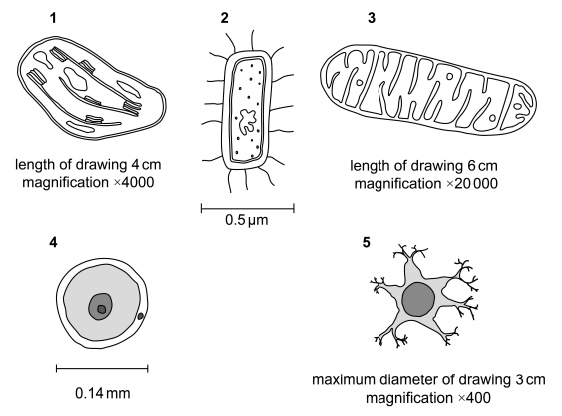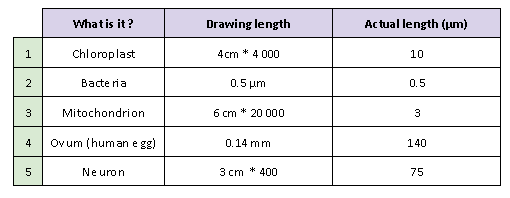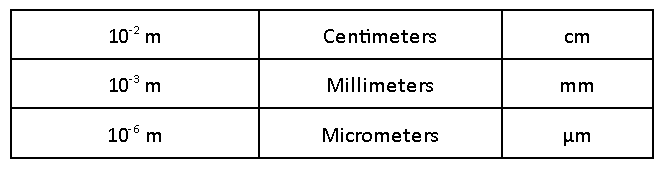# IMAT 2020 Q40 [Microscopic Structures]

The diagrams show five different microscopic structures.

The structures are not drawn to the same scale.

Which of these structures is the smallest?A. 4
B. 5
C. 1
D. 3
E. 2

The correct answer is E.

To answer this question, there are 2 steps :

1. First, we have to determine the actual size of each structure : they are all magnified by a certain number of times, which means that the actual sizes are divided by that number.

2. Then, we have to put each structure to the same scale : we will put all of them into micrometers (µm)Based on these elements, we can determine that row 2 (bacteria) is the smallest.

The trick here is to be able to navigate through all the different units with ease :Therefore :

• 1 cm = 10 000 µm
• 1 mm = 1 000 µm.
2 Likes